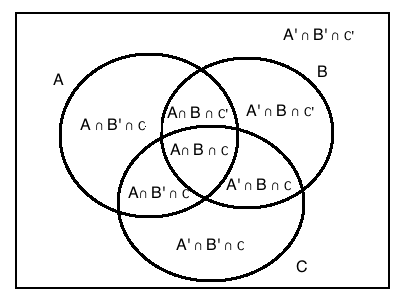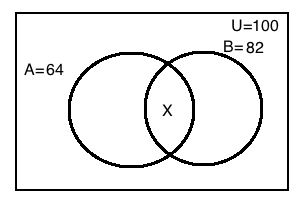Venn Diagrams

Theory

Undoubtedly one of the easiest parts of CAT. Most of the formulae in this section can be deduced logically with little effort. The difficult part of the problem is translating the sentences into areas of the venn diagram. While solving, pay careful attention to phrases like and, or, not, only, in as these generally signify the relationship.

Formula

A is a subset of B if and only if all elements of  A are already present in B

• The set of all subsets of a set A is called the power set of A

• The null set is a subset of all sets

• If A=B, A $$\subset$$ B and B $$\subset$$ A

• A $$\cup$$ (B$$\cup$$C) = (A $$\cup$$ B)$$\cup$$C
• A $$\cap$$ (B$$\cap$$C) = (A $$\cap$$ B)$$\cap$$C
• A $$\cup$$ (B$$\cap$$C) = (A $$\cup$$ B)$$\cap$$( A $$\cup$$ C)
• A $$\cap$$ (B$$\cup$$C) = (A $$\cap$$ B)$$\cup$$( A $$\cap$$ C)
Formula• Consider three intersecting sets A, B and C. A represents all the elements in set A and A’ represents all the elements not in A. The image shows the different areas in a venn diagram and the meaning of each area.* $$n(A \cup B)$$ = $$n(A)+n(B)-n(A \cap B)$$
• $$n(A \cup B \cup C)$$ = $$n(A)+n(B)+n(C)$$-$$n(A \cap B)$$ - $$n(B \cap C)$$ - $$n(A \cap C)$$ + $$n(A \cap B \cap C)$$
• Only A can be translated as A and not B and not C
• Similarly, A’ and B’ and C’  = Universal set – (A or B or C)
Theory
• Maxima and minima of overlap: As shown in the image, what is the maximum and minimum value of X?• To maximize X, we must assume that for the smaller set A, A and B’=0. Hence all of A’s 64 elements are in A and B. Therefore max of X=64
• To minimize X, we must assume that the union of A and B is as large as possible. In this case, A or B=100. Hence, n(A or B)=n(A)+n(B)-n(A and B). Hence n(A and B)=64+82-100=46.
Theory

The similar concept applies for venn diagrams with three sets. Remember that,

To maximize overlap,

• Union should be as small as possible
• Calculate the surplus = n( A) +n(B) +n(C)-n(A or B or C)
• This can be attributed to $$n(A\cap B\cap C')$$, $$n(A\cap B'\cap C)$$, $$n(A'\cap B\cap C)$$, $$n(A\cap B\cap C)$$. To maximize the overlap, set the other three terms to zero.

To minimize overlap,

• Union should be as large as possible
• Calculate the surplus = n( A) +n(B) +n(C)-n(A or B or C)
• This can be attributed to $$n(A\cap B\cap C')$$, $$n(A\cap B'\cap C)$$, $$n(A'\cap B\cap C)$$, $$n(A\cap B\cap C)$$. To minimize the overlap, set the other three terms to maximum possible.